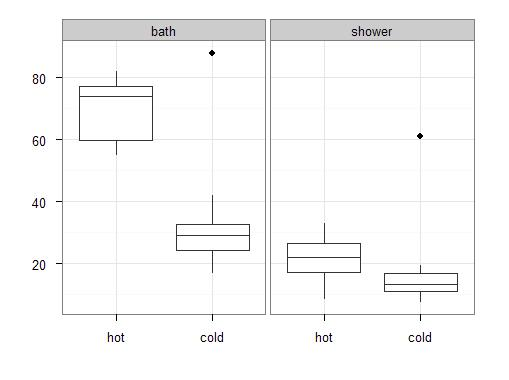## 2011-12-29

### How much is a shower?

After looking at my heating expenses, I turned to the costs for water heating. For some time, I looked at my water meter before and after taking a shower or a bath. Quite often, I forgot one or the other measurement, but I collected about 40 observations. Here is what they look like:The data suggest that for a shower, it takes between 17 and 26.5 liters hot water and between 11 and 16.5 liters cold water. For a bath, it is 60 to 77 liters hot water and 24 to 32.5 liters cold water. (The numbers refer to the 25% and 75% percentiles, respectively.) The larger share of cold water for a shower makes sense, since I use cold water at the end of the shower for its “invigorating effect”.

Multiplied with the average costs, as charged by my landlord the last three years, a bath takes 0.94 to 1.22 EUR and a shower costs 0.29 to 0.45 EUR (again, first and third quartiles). So taking a shower for 0.50 EUR at the fitness club is not optimal, but also not very expensive.

There are water saving shower heads for 30 EUR. It is advertised that such a shower head uses 6.5 liter per minute instead the usual 15 to 16 liters per minute. I believe 15 liters per minute is too much. So let’s assume I save 3.5 liters per minute or (using the median water use of the data) 12 liters per shower. Is it cost-efficient?

Twelve liters less per shower at 10.5 EUR/cbm means a saving of 0.126 EUR per shower. I assume 20 showers a month. This is tentative, since with this assumption the costs sum up to 70 EUR a year for hot water while my bills amount in average to 110 EUR total costs for hot water. So the new shower head saves 20*0.126=2.52 EUR per month.

Let’s calculate the payback period. With an interest rate of 4% p.a. and 5 years expected serviceable life the monthly gain calculates to

2. 52 - 30 * 0. 04 / 12 - 30 / (5 * 12) = 1. 92

so after 30 / 1. 92 = 16 months, the investment is repaid. This seems acceptable. But just to be sure, let’s calculate the internal rate of return, too. In excel, there is a function `IRR` for this procedure, which is implemented in three lines in R. For convenience, the `irr` function is stored in the `pft` package. The result is 8.3% which seems decent.

Just for reference, here comes the R code for the plot and the `irr` function:

The water usage dataset and `irr` function is stored in the `pft` dataset. The package is not on CRAN yet, because I consider it not mature enough. To install the package, specify my private repository:

``````> install.packages(
+   "pft",
+   repos=c(getOption("repos"),
+      "http://userpage.fu-berlin.de/~kweinert/R"),
+   dependencies=c("Depends", "Suggests")
+ )``````

To produce the plot, the `ggplot2` package is required. The chart is then produced thus:

``````> library(pft)
> library(ggplot2)
> g <- melt(pft.water[,c("date", "bath.shower", "warm.used", "cold.used")], id.vars=c("date", "bath.shower"), measure.vars=c("warm.used", "cold.used"), na.rm=TRUE)
> levels(g\$bath.shower) <- c("bath", "shower")
> levels(g\$variable) <- c("hot", "cold")
> g\$value <- g\$value * 1000.
> p <- ggplot(g, aes(variable, value)) +
+       geom_boxplot() +
+       facet_wrap(~ bath.shower) +
+       labs(x="", y="") +
+       theme_bw()
> print(p)``````

The calculation of water use and costs was a bit convoluted. Here is the code to calculate the 25%, 50% and 75% percentiles of water use (the numbers refer to liters):

``````> use <- ddply(g, .(bath.shower, variable), function(df) quantile(df\$value, probs=c(0.25,0.5,0.75)))
> colnames(use) <- c("bs", "hc", "q1", "q2", "q3")
> use
bs   hc     q1    q2     q3
1   bath  hot 59.750 74.00 77.200
2   bath cold 24.250 29.10 32.500
3 shower  hot 17.100 21.80 26.550
4 shower cold 10.875 13.15 16.625``````

And here is how to calculate the costs (numbers refer to EUR):

``````> use <- melt(use, id.vars=c("bs", "hc"), measure.vars=c("q1", "q2", "q3"))
> use <- transform(use, eur=ifelse(hc=="hot",value*13.274398, value*6.006333)/1000.)
> use\$value <- NULL
> use <- ddply(use, .(bs, variable), function(df) sum(df\$eur))
> use <- cast(use, bs ~ variable, value="V1")
> use
bs        q1        q2        q3
1   bath 0.9387989 1.1570897 1.2199893
2 shower 0.2923111 0.3683652 0.4522906``````

The `irr` function is really three lines long. Here is how to calculate the internal rate of return for the shower head:

``````> irr
function (x, start = 0.1)
{
t <- seq_along(x) - 1
f <- function(i) abs(sum(x/(1 + i)^t))
return(nlm(f, start)\$estimate)
}
<environment: namespace:pft>
> x <- c(-30, rep(2.52, 12*5))
> irr(x)
 0.08330924``````